# Mean State

Period Mean (original grids) [Watt m-2]
Model Period Mean (intersection) [Watt m-2]
Model Period Mean (complement) [Watt m-2]
Benchmark Period Mean (intersection) [Watt m-2]
Benchmark Period Mean (complement) [Watt m-2]
Bias [Watt m-2]
RMSE [Watt m-2]
Phase Shift [months]
Bias Score 
RMSE Score 
Seasonal Cycle Score 
Spatial Distribution Score 
Interannual Variability Score 
Overall Score 
Benchmark [-] -56.8
CLM4 [-] -61.2 -61.0 0.00 -57.0 -52.3 -4.14 13.3 1.69 0.63 0.46 0.76 0.99 0.81 0.69
CLM4.5 [-] -65.3 -65.5 0.00 -57.0 -52.3 -8.33 16.2 1.70 0.50 0.46 0.76 0.99 0.83 0.66
CLM5 [-] -66.5 -66.5 0.00 -57.0 -52.3 -9.56 16.5 1.67 0.44 0.46 0.76 0.97 0.82 0.65
Period Mean (original grids) [Watt m-2]
Model Period Mean (intersection) [Watt m-2]
Model Period Mean (complement) [Watt m-2]
Benchmark Period Mean (intersection) [Watt m-2]
Benchmark Period Mean (complement) [Watt m-2]
Bias [Watt m-2]
RMSE [Watt m-2]
Phase Shift [months]
Bias Score 
RMSE Score 
Seasonal Cycle Score 
Spatial Distribution Score 
Interannual Variability Score 
Overall Score 
Benchmark [-] -87.0
CLM4 [-] -90.3 -91.1 0.00 -87.4 -58.4 -3.32 18.8 0.274 0.78 0.59 0.97 0.95 0.74 0.77
CLM4.5 [-] -95.9 -96.5 0.00 -87.4 -58.4 -8.68 21.6 0.279 0.68 0.60 0.98 0.75 0.75 0.73
CLM5 [-] -97.3 -98.0 0.00 -87.4 -58.4 -10.2 22.1 0.309 0.68 0.59 0.97 0.81 0.72 0.73
Period Mean (original grids) [Watt m-2]
Model Period Mean (intersection) [Watt m-2]
Model Period Mean (complement) [Watt m-2]
Benchmark Period Mean (intersection) [Watt m-2]
Benchmark Period Mean (complement) [Watt m-2]
Bias [Watt m-2]
RMSE [Watt m-2]
Phase Shift [months]
Bias Score 
RMSE Score 
Seasonal Cycle Score 
Spatial Distribution Score 
Interannual Variability Score 
Overall Score 
Benchmark [-] -81.4
CLM4 [-] -86.5 -86.6 0.00 -82.3 -62.6 -2.69 17.2 0.810 0.70 0.52 0.91 0.99 0.73 0.73
CLM4.5 [-] -92.8 -92.9 0.00 -82.3 -62.6 -8.33 20.0 0.761 0.59 0.52 0.91 0.97 0.74 0.71
CLM5 [-] -91.9 -92.0 0.00 -82.3 -62.6 -7.58 19.7 0.810 0.62 0.51 0.90 0.97 0.71 0.70
Period Mean (original grids) [Watt m-2]
Model Period Mean (intersection) [Watt m-2]
Model Period Mean (complement) [Watt m-2]
Benchmark Period Mean (intersection) [Watt m-2]
Benchmark Period Mean (complement) [Watt m-2]
Bias [Watt m-2]
RMSE [Watt m-2]
Phase Shift [months]
Bias Score 
RMSE Score 
Seasonal Cycle Score 
Spatial Distribution Score 
Interannual Variability Score 
Overall Score 
Benchmark [-] -37.2
CLM4 [-] -37.6 -37.7 0.00 -36.9 -41.0 -1.32 15.3 1.10 0.75 0.42 0.86 0.99 0.87 0.72
CLM4.5 [-] -37.6 -37.7 0.00 -36.9 -41.0 -1.27 15.4 1.06 0.75 0.42 0.87 0.99 0.87 0.72
CLM5 [-] -39.5 -39.6 0.00 -36.9 -41.0 -3.38 16.5 1.11 0.72 0.40 0.86 0.97 0.88 0.70
Period Mean (original grids) [Watt m-2]
Model Period Mean (intersection) [Watt m-2]
Model Period Mean (complement) [Watt m-2]
Benchmark Period Mean (intersection) [Watt m-2]
Benchmark Period Mean (complement) [Watt m-2]
Bias [Watt m-2]
RMSE [Watt m-2]
Phase Shift [months]
Bias Score 
RMSE Score 
Seasonal Cycle Score 
Spatial Distribution Score 
Interannual Variability Score 
Overall Score 
Benchmark [-] -44.6
CLM4 [-] -42.9 -42.8 0.00 -43.2 -49.2 0.326 8.66 1.94 0.43 0.35 0.70 0.97 0.79 0.60
CLM4.5 [-] -45.3 -45.3 0.00 -43.2 -49.2 -1.44 9.30 1.94 0.40 0.35 0.70 0.95 0.79 0.59
CLM5 [-] -49.7 -49.7 0.00 -43.2 -49.2 -5.81 10.4 1.88 0.35 0.34 0.72 0.98 0.78 0.59
Period Mean (original grids) [Watt m-2]
Model Period Mean (intersection) [Watt m-2]
Model Period Mean (complement) [Watt m-2]
Benchmark Period Mean (intersection) [Watt m-2]
Benchmark Period Mean (complement) [Watt m-2]
Bias [Watt m-2]
RMSE [Watt m-2]
Phase Shift [months]
Bias Score 
RMSE Score 
Seasonal Cycle Score 
Spatial Distribution Score 
Interannual Variability Score 
Overall Score 
Benchmark [-] -42.1
CLM4 [-] -48.7 -48.7 0.00 -41.9 -51.7 -6.70 13.6 0.597 0.65 0.50 0.93 0.96 0.91 0.74
CLM4.5 [-] -49.0 -49.0 0.00 -41.9 -51.7 -7.00 13.7 0.661 0.64 0.50 0.92 1.0 0.91 0.74
CLM5 [-] -52.1 -52.1 0.00 -41.9 -51.7 -10.1 15.7 0.716 0.54 0.49 0.92 0.99 0.89 0.72
Period Mean (original grids) [Watt m-2]
Model Period Mean (intersection) [Watt m-2]
Model Period Mean (complement) [Watt m-2]
Benchmark Period Mean (intersection) [Watt m-2]
Benchmark Period Mean (complement) [Watt m-2]
Bias [Watt m-2]
RMSE [Watt m-2]
Phase Shift [months]
Bias Score 
RMSE Score 
Seasonal Cycle Score 
Spatial Distribution Score 
Interannual Variability Score 
Overall Score 
Benchmark [-] -67.1
CLM4 [-] -69.3 -69.0 0.00 -67.3 -59.4 -0.910 15.2 0.947 0.73 0.51 0.87 1.0 0.81 0.74
CLM4.5 [-] -69.8 -69.5 0.00 -67.3 -59.4 -1.37 15.1 0.952 0.73 0.51 0.87 1.0 0.82 0.74
CLM5 [-] -72.9 -72.6 0.00 -67.3 -59.4 -4.47 16.3 1.02 0.68 0.50 0.85 0.99 0.81 0.72
Period Mean (original grids) [Watt m-2]
Model Period Mean (intersection) [Watt m-2]
Model Period Mean (complement) [Watt m-2]
Benchmark Period Mean (intersection) [Watt m-2]
Benchmark Period Mean (complement) [Watt m-2]
Bias [Watt m-2]
RMSE [Watt m-2]
Phase Shift [months]
Bias Score 
RMSE Score 
Seasonal Cycle Score 
Spatial Distribution Score 
Interannual Variability Score 
Overall Score 
Benchmark [-] -71.2
CLM4 [-] -74.0 -74.3 0.00 -72.6 -53.4 0.779 17.0 0.932 0.73 0.55 0.88 0.98 0.72 0.73
CLM4.5 [-] -78.6 -78.8 0.00 -72.6 -53.4 -3.27 17.9 0.875 0.69 0.55 0.89 0.97 0.73 0.73
CLM5 [-] -80.3 -80.5 0.00 -72.6 -53.4 -5.12 18.3 0.861 0.68 0.55 0.89 0.95 0.71 0.72
Period Mean (original grids) [Watt m-2]
Model Period Mean (intersection) [Watt m-2]
Model Period Mean (complement) [Watt m-2]
Benchmark Period Mean (intersection) [Watt m-2]
Benchmark Period Mean (complement) [Watt m-2]
Bias [Watt m-2]
RMSE [Watt m-2]
Phase Shift [months]
Bias Score 
RMSE Score 
Seasonal Cycle Score 
Spatial Distribution Score 
Interannual Variability Score 
Overall Score 
Benchmark [-] -31.1
CLM4 [-] -37.1 -37.2 0.00 -31.1 -32.1 -6.10 14.5 0.706 0.66 0.46 0.93 0.97 0.89 0.73
CLM4.5 [-] -36.7 -36.7 0.00 -31.1 -32.1 -5.59 14.2 0.749 0.68 0.46 0.93 0.99 0.89 0.73
CLM5 [-] -38.9 -39.0 0.00 -31.1 -32.1 -7.87 16.1 0.817 0.61 0.43 0.92 0.96 0.90 0.71
Period Mean (original grids) [Watt m-2]
Model Period Mean (intersection) [Watt m-2]
Model Period Mean (complement) [Watt m-2]
Benchmark Period Mean (intersection) [Watt m-2]
Benchmark Period Mean (complement) [Watt m-2]
Bias [Watt m-2]
RMSE [Watt m-2]
Phase Shift [months]
Bias Score 
RMSE Score 
Seasonal Cycle Score 
Spatial Distribution Score 
Interannual Variability Score 
Overall Score 
Benchmark [-] -96.8
CLM4 [-] -97.2 -97.2 0.00 -99.4 -63.9 5.29 15.7 0.476 0.67 0.56 0.93 0.96 0.77 0.74
CLM4.5 [-] -97.8 -97.8 0.00 -99.4 -63.9 4.73 15.8 0.485 0.67 0.55 0.93 0.96 0.77 0.74
CLM5 [-] -99.6 -99.6 0.00 -99.4 -63.9 3.14 15.8 0.545 0.69 0.54 0.92 0.96 0.74 0.73
Period Mean (original grids) [Watt m-2]
Model Period Mean (intersection) [Watt m-2]
Model Period Mean (complement) [Watt m-2]
Benchmark Period Mean (intersection) [Watt m-2]
Benchmark Period Mean (complement) [Watt m-2]
Bias [Watt m-2]
RMSE [Watt m-2]
Phase Shift [months]
Bias Score 
RMSE Score 
Seasonal Cycle Score 
Spatial Distribution Score 
Interannual Variability Score 
Overall Score 
Benchmark [-] -55.6
CLM4 [-] -55.4 -55.4 0.00 -55.1 -60.8 -1.04 11.3 0.805 0.70 0.47 0.90 0.98 0.88 0.73
CLM4.5 [-] -54.9 -55.0 0.00 -55.1 -60.8 -0.517 10.7 0.784 0.73 0.48 0.90 0.98 0.88 0.74
CLM5 [-] -58.2 -58.2 0.00 -55.1 -60.8 -3.61 11.6 0.745 0.65 0.47 0.91 0.97 0.87 0.72
Period Mean (original grids) [Watt m-2]
Model Period Mean (intersection) [Watt m-2]
Model Period Mean (complement) [Watt m-2]
Benchmark Period Mean (intersection) [Watt m-2]
Benchmark Period Mean (complement) [Watt m-2]
Bias [Watt m-2]
RMSE [Watt m-2]
Phase Shift [months]
Bias Score 
RMSE Score 
Seasonal Cycle Score 
Spatial Distribution Score 
Interannual Variability Score 
Overall Score 
Benchmark [-] -52.6
CLM4 [-] -67.1 -67.1 0.00 -65.2 -46.5 -0.870 15.8 0.957 0.65 0.50 0.87 0.95 0.77 0.71
CLM4.5 [-] -68.7 -68.7 0.00 -65.2 -46.5 -2.28 16.3 0.939 0.62 0.50 0.87 0.96 0.78 0.70
CLM5 [-] -71.2 -71.2 0.00 -65.2 -46.5 -4.70 17.3 0.974 0.58 0.49 0.87 0.94 0.77 0.69
Period Mean (original grids) [Watt m-2]
Model Period Mean (intersection) [Watt m-2]
Model Period Mean (complement) [Watt m-2]
Benchmark Period Mean (intersection) [Watt m-2]
Benchmark Period Mean (complement) [Watt m-2]
Bias [Watt m-2]
RMSE [Watt m-2]
Phase Shift [months]
Bias Score 
RMSE Score 
Seasonal Cycle Score 
Spatial Distribution Score 
Interannual Variability Score 
Overall Score 
Benchmark [-] -121.
CLM4 [-] -106. -106. 0.00 -122. -81.3 17.1 26.5 0.652 0.54 0.54 0.91 0.83 0.63 0.67
CLM4.5 [-] -106. -106. 0.00 -122. -81.3 16.7 26.3 0.644 0.54 0.54 0.91 0.84 0.64 0.67
CLM5 [-] -108. -108. 0.00 -122. -81.3 15.4 25.9 0.675 0.56 0.53 0.91 0.82 0.64 0.66
Period Mean (original grids) [Watt m-2]
Model Period Mean (intersection) [Watt m-2]
Model Period Mean (complement) [Watt m-2]
Benchmark Period Mean (intersection) [Watt m-2]
Benchmark Period Mean (complement) [Watt m-2]
Bias [Watt m-2]
RMSE [Watt m-2]
Phase Shift [months]
Bias Score 
RMSE Score 
Seasonal Cycle Score 
Spatial Distribution Score 
Interannual Variability Score 
Overall Score 
Benchmark [-] -34.3
CLM4 [-] -39.8 -39.8 0.00 -34.2 -36.5 -5.83 13.4 0.708 0.66 0.47 0.91 0.90 0.87 0.71
CLM4.5 [-] -39.5 -39.5 0.00 -34.2 -36.5 -5.53 13.4 0.678 0.67 0.47 0.92 0.93 0.87 0.72
CLM5 [-] -42.1 -42.1 0.00 -34.2 -36.5 -8.40 15.3 0.806 0.58 0.45 0.89 0.84 0.88 0.68
Period Mean (original grids) [Watt m-2]
Model Period Mean (intersection) [Watt m-2]
Model Period Mean (complement) [Watt m-2]
Benchmark Period Mean (intersection) [Watt m-2]
Benchmark Period Mean (complement) [Watt m-2]
Bias [Watt m-2]
RMSE [Watt m-2]
Phase Shift [months]
Bias Score 
RMSE Score 
Seasonal Cycle Score 
Spatial Distribution Score 
Interannual Variability Score 
Overall Score 
Benchmark [-] -70.7
CLM4 [-] -83.1 -83.1 0.00 -73.4 -57.5 -6.98 16.2 1.10 0.54 0.48 0.83 0.99 0.81 0.69
CLM4.5 [-] -83.0 -83.0 0.00 -73.4 -57.5 -6.32 15.6 1.05 0.57 0.48 0.84 0.99 0.81 0.70
CLM5 [-] -84.6 -84.6 0.00 -73.4 -57.5 -7.82 16.0 1.09 0.53 0.48 0.83 1.0 0.80 0.69
Period Mean (original grids) [Watt m-2]
Model Period Mean (intersection) [Watt m-2]
Model Period Mean (complement) [Watt m-2]
Benchmark Period Mean (intersection) [Watt m-2]
Benchmark Period Mean (complement) [Watt m-2]
Bias [Watt m-2]
RMSE [Watt m-2]
Phase Shift [months]
Bias Score 
RMSE Score 
Seasonal Cycle Score 
Spatial Distribution Score 
Interannual Variability Score 
Overall Score 
Benchmark [-] -53.2
CLM4 [-] -55.8 -55.6 0.00 -52.9 -58.5 -2.69 10.1 0.852 0.75 0.47 0.87 1.0 0.91 0.74
CLM4.5 [-] -57.7 -57.6 0.00 -52.9 -58.5 -4.42 10.9 0.695 0.67 0.47 0.89 1.0 0.90 0.73
CLM5 [-] -61.8 -61.7 0.00 -52.9 -58.5 -8.67 13.0 0.738 0.50 0.47 0.88 0.99 0.89 0.70
Period Mean (original grids) [Watt m-2]
Model Period Mean (intersection) [Watt m-2]
Model Period Mean (complement) [Watt m-2]
Benchmark Period Mean (intersection) [Watt m-2]
Benchmark Period Mean (complement) [Watt m-2]
Bias [Watt m-2]
RMSE [Watt m-2]
Phase Shift [months]
Bias Score 
RMSE Score 
Seasonal Cycle Score 
Spatial Distribution Score 
Interannual Variability Score 
Overall Score 
Benchmark [-] -42.5
CLM4 [-] -49.6 -49.6 0.00 -42.4 -45.4 -7.03 13.0 0.533 0.66 0.53 0.94 0.97 0.88 0.75
CLM4.5 [-] -49.6 -49.6 0.00 -42.4 -45.4 -7.01 13.2 0.548 0.66 0.52 0.94 0.98 0.88 0.75
CLM5 [-] -52.8 -52.8 0.00 -42.4 -45.4 -10.2 15.3 0.536 0.55 0.51 0.94 0.98 0.86 0.73
Period Mean (original grids) [Watt m-2]
Model Period Mean (intersection) [Watt m-2]
Model Period Mean (complement) [Watt m-2]
Benchmark Period Mean (intersection) [Watt m-2]
Benchmark Period Mean (complement) [Watt m-2]
Bias [Watt m-2]
RMSE [Watt m-2]
Phase Shift [months]
Bias Score 
RMSE Score 
Seasonal Cycle Score 
Spatial Distribution Score 
Interannual Variability Score 
Overall Score 
Benchmark [-] -80.3
CLM4 [-] -83.4 -83.3 0.00 -80.8 -54.9 -2.19 16.9 0.276 0.76 0.57 0.97 0.94 0.78 0.77
CLM4.5 [-] -83.8 -83.6 0.00 -80.8 -54.9 -2.45 16.3 0.246 0.76 0.59 0.97 0.95 0.79 0.77
CLM5 [-] -86.8 -86.6 0.00 -80.8 -54.9 -5.44 18.1 0.247 0.72 0.57 0.97 0.94 0.77 0.76
Period Mean (original grids) [Watt m-2]
Model Period Mean (intersection) [Watt m-2]
Model Period Mean (complement) [Watt m-2]
Benchmark Period Mean (intersection) [Watt m-2]
Benchmark Period Mean (complement) [Watt m-2]
Bias [Watt m-2]
RMSE [Watt m-2]
Phase Shift [months]
Bias Score 
RMSE Score 
Seasonal Cycle Score 
Spatial Distribution Score 
Interannual Variability Score 
Overall Score 
Benchmark [-] -43.7
CLM4 [-] -47.2 -47.1 0.00 -43.6 -47.7 -3.81 11.2 1.71 0.62 0.46 0.76 0.82 0.74 0.64
CLM4.5 [-] -50.3 -50.1 0.00 -43.6 -47.7 -6.50 12.5 1.66 0.53 0.46 0.77 0.77 0.75 0.62
CLM5 [-] -51.8 -51.7 0.00 -43.6 -47.7 -8.13 13.1 1.56 0.46 0.46 0.79 0.87 0.75 0.63
Period Mean (original grids) [Watt m-2]
Model Period Mean (intersection) [Watt m-2]
Model Period Mean (complement) [Watt m-2]
Benchmark Period Mean (intersection) [Watt m-2]
Benchmark Period Mean (complement) [Watt m-2]
Bias [Watt m-2]
RMSE [Watt m-2]
Phase Shift [months]
Bias Score 
RMSE Score 
Seasonal Cycle Score 
Spatial Distribution Score 
Interannual Variability Score 
Overall Score 
Benchmark [-] -64.5
CLM4 [-] -67.2 -67.7 0.00 -64.8 -56.3 -2.38 14.2 0.894 0.66 0.47 0.88 0.86 0.82 0.69
CLM4.5 [-] -70.0 -70.5 0.00 -64.8 -56.3 -4.94 14.8 0.875 0.65 0.47 0.88 0.85 0.83 0.69
CLM5 [-] -72.2 -72.6 0.00 -64.8 -56.3 -7.17 14.8 0.801 0.65 0.48 0.90 0.90 0.81 0.70
Period Mean (original grids) [Watt m-2]
Model Period Mean (intersection) [Watt m-2]
Model Period Mean (complement) [Watt m-2]
Benchmark Period Mean (intersection) [Watt m-2]
Benchmark Period Mean (complement) [Watt m-2]
Bias [Watt m-2]
RMSE [Watt m-2]
Phase Shift [months]
Bias Score 
RMSE Score 
Seasonal Cycle Score 
Spatial Distribution Score 
Interannual Variability Score 
Overall Score 
Benchmark [-] -80.0
CLM4 [-] -82.7 -82.2 0.00 -83.0 -54.0 3.20 20.0 0.766 0.56 0.44 0.89 0.90 0.78 0.67
CLM4.5 [-] -84.6 -84.1 0.00 -83.0 -54.0 1.53 20.0 0.799 0.55 0.44 0.88 0.91 0.78 0.67
CLM5 [-] -85.7 -85.3 0.00 -83.0 -54.0 0.359 19.8 0.785 0.54 0.44 0.89 0.89 0.77 0.66

# Temporally integrated period mean

BENCHMARK MEAN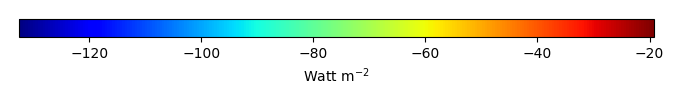MODEL MEANBIAS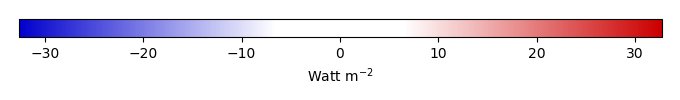BIAS SCORERMSE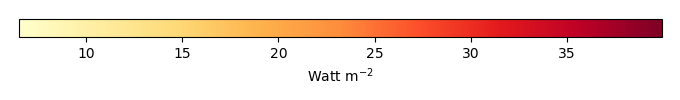RMSE SCOREBENCHMARK INTERANNUAL VARIABILITY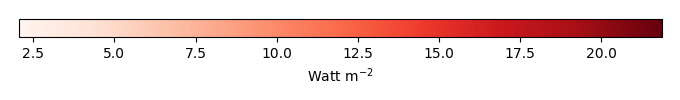MODEL INTERANNUAL VARIABILITYINTERANNUAL VARIABILITY SCOREBENCHMARK MAX MONTHMODEL MAX MONTHDIFFERENCE IN MAX MONTH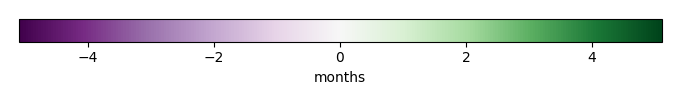SEASONAL CYCLE SCORESPATIAL TAYLOR DIAGRAMMODEL COLORS# Spatially integrated regional mean

MODEL COLORSREGIONAL MEANANNUAL CYCLEMONTHLY ANOMALYANNUAL CYCLE# All Models

BenchmarkCLM4CLM4.5CLM5# Data Information

creation_date: Tue Jul 1 08:25:00 PDT 2014

source_file: This product is generated from monthly 1 degree GEWEX SRB Radiation observations